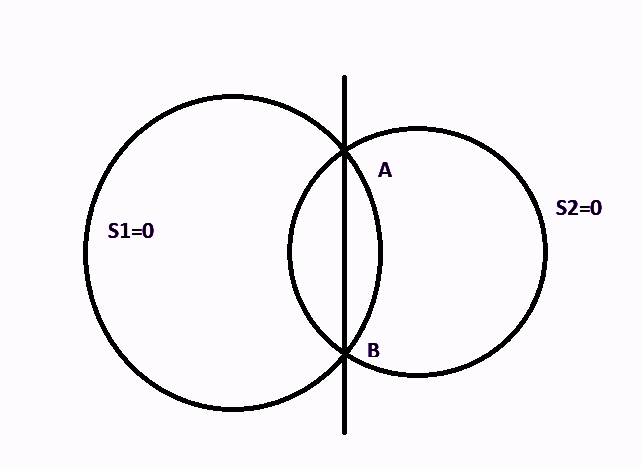QUESTION

# Find the general equation of all circles, so that the radical axis of any two of them is the same as those of the circles ${x^2} + {y^2} = 4$ and ${x^2} + {y^2} + 2x + 4y - 6 = 0$.

Hint: This type of question based on intersection of circles is done using the concept of coaxial systems of circles. In coaxial systems of circles, the chord or the radical axis are the same. In a coaxial system, two circles intersect at point A and B, then AB will be the radical axis. For all circles which pass through the points A and B, all circles will have the same radical axis, i.e. line AB.According to question, we have to form a general equation of all circles, so that radical axis is same as that of for the circles ${S_1} \Rightarrow {x^2} + {y^2} - 4 = 0$ and ${S_2} \Rightarrow {x^2} + {y^2} + 2x + 4y - 6 = 0$. Let A and B be the intersection point of these two circles.

The general equation for such circle’s is given by ${S_1} + \lambda {S_2} = 0$.
$\Rightarrow ({x^2} + {y^2} - 4) + \lambda ({x^2} + {y^2} + 2x + 4y - 6) = 0$

Hence, this is the general equation of all circles which will pass through the intersection of the circles ${x^2} + {y^2} = 4$ and ${x^2} + {y^2} + 2x + 4y - 6 = 0$. This system is called a coaxial system of circles as their common chord or radical axis is the line AB.

Note- We can also get the equation of common chord AB, $\therefore {S_1} - {S_2} = 0$. Let ${S_1} - {S_2} = 0 \Rightarrow P = 0$. Then ${S_1} + \lambda P = 0$ represents the equation of the family of circles which passes through the intersection of ${S_1} = 0$ and ${S_2} = 0$. We will get $P = {S_1} - {S_2} \Rightarrow - 2x - 4y + 2 = 0$or $P \Rightarrow x + 2y - 1 = 0$.
$\therefore$Required family of circles is given by ${S_1} + \lambda P = 0$, i.e. $({x^2} + {y^2} - 4) + \lambda (x + 2y - 1) = 0$.